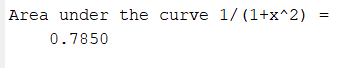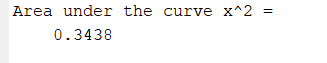# MATLAB – Trapezoidal numerical integration without using trapz

• Difficulty Level : Medium
• Last Updated : 04 Jul, 2021

Trapezoidal rule is utilized to discover the approximation of a definite integral. The main idea in the Trapezoidal rule is to accept the region under the graph of the given function to be a trapezoid rather than a rectangle shape and calculate its region.

The formula for numerical integration using trapezoidal rule is:where h = (b-a)/n

Now we take an example for calculating the area under the curveusing 10 subintervals.

Example:

## Matlab

 `% MATLAB program for calculate the ``% area under the curve ∫_0^11/(1+x^2) dx ``% using 10 subintervals specify the variable``% x as symbolic ones The syms function creates ``% a variable dynamically and automatically assigns``% to a MATLAB variable with the same name``syms x`` ` `% Lower Limit``a=0;  `` ` `% Upper Limit``b=1;   `` ` `% Number of segments``n=10; `` ` `% Declare the function``f1=1/(1+x^2);`` ` `% inline creates a function of``% string containing in f1``f=inline(f1); `` ` `% h is the segment size``h=(b - a)/n;`` ` `% X stores the summation of first``% and last segment``X=f(a)+f(b);`` ` `% variable R stores the summation of``% all the terms from 1 to n-1``R=0;``for` `i = 1:1:n-1``    ``xi=a+(i*h);``    ``R=R+f(xi);``end`` ` `% Formula to calculate numerical integration``% using Trapezoidal Rule``I=(h/2)*(X+2*R);`` ` `% Display the output``disp(``'Area under the curve 1/(1+x^2) = '``);``disp(I);`

Output:Let’s take another example for calculating the area under the curveusing 4 subintervals.

Example:

## Matlab

 `% MATLAB program for calculate``% the area under the curve∫_0^1x^2 dx    ``% using 4 subintervals.``% specify the variable x as symbolic ones`` ` `syms x`` ` `% Lower Limit``a=0; `` ` `% Upper Limit``b=1; `` ` `% Number of segments``n=4;  `` ` `% Declare the function``f1=x^2;`` ` `% inline creates a function of``% string containing in f1``f=inline(f1); `` ` `% h is the segment size``h=(b - a)/n;``X=f(a)+f(b);`` ` `% variable R stores the summation ``% of all the terms from 1 to n-1``R=0;``for` `i = 1:1:n-1``    ``xi=a+(i*h);``    ``R=R+f(xi);``end`` ` `% Formula to calculate numerical``% integration using Trapezoidal Rule``I=(h/2)*(X+2*R);`` ` `% Display the output``disp(``'Area under the curve x^2 = '``);``disp(I);`

Output:My Personal Notes arrow_drop_up